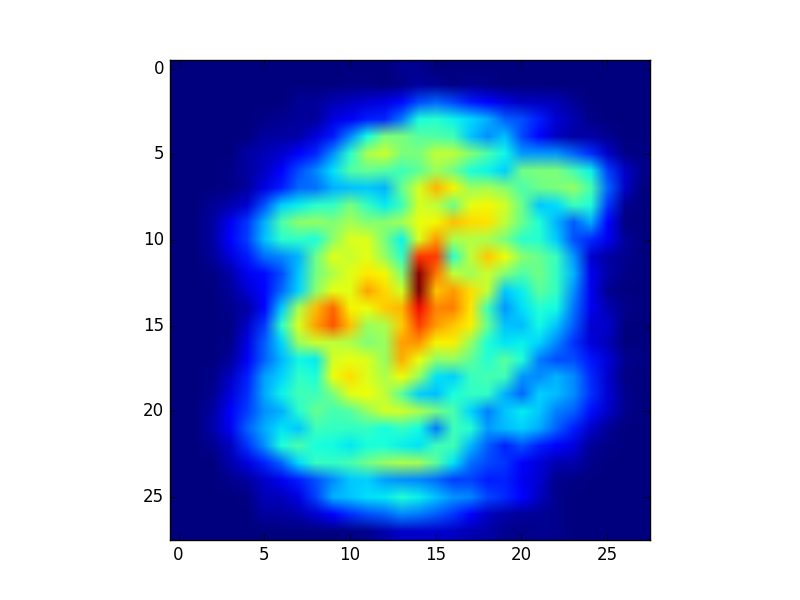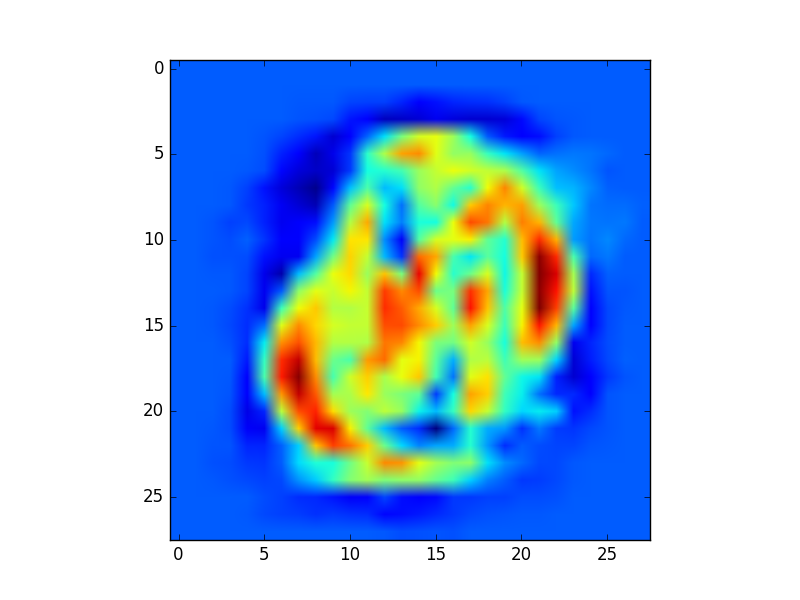# Impact of inverting grayscale values on mnist dataset

http://yann.lecun.com/exdb/mnist/

Pixel values are 0 to 255. 0 means background (white), 255 means foreground (black).

Is there a reason why the original mnist sets the background to a low value (0) and the foreground to the highest value (255) ?

Can inverting those values have an impact on the performences when training on the same convnet ? (does this comment makes sense)

Or is it just to speed up the training, as most of the image is set to 0 on mnist characters?

• Where in the dataset are "black" and "white" even referenced? You appear to be referring to a particular visualization of the contents of the dataset, not the contents themselves.
– whuber
Jun 22, 2016 at 15:39
• The mnist description mentions black and white. Is there any différence if I train with a dataset where pixels of characters (forms we try to learn) have the highest value or lowest ? Jun 22, 2016 at 15:47
• Thank you for the link. "Black" and "white" are defined there as "foreground" and "background," respectively. (I see you just included that fact in an edit.) Because the latter do have distinct meanings, and because the images are actually grayscale (with values ranging from 0 to 255), any nonlinear transformations of those values potentially depend on how foreground and background are encoded. Therefore the question might not be as entirely trivial as suggested in an earlier (now deleted) comment.
– whuber
Jun 22, 2016 at 16:02
• to my understanding, theoretically there should be no difference, as long as your model's first layer has bias, or is followed by a BN layer. The reason is that the inversion function f(x) = 255 - x = -x + 255 is linear. This means that given a model that works on the original mnist images, you can manually tweak the learned weights of the first layer to give identical results on inverted images, buy stacking the learned linear function with the inversion linear function into a single linear function. This means that theoretically the tweaked weights could have been learned while training. May 7 at 9:01

Here's a quick test on the mnist_softmax implemention from the tensorflow tutorial. You can append this code at the end of the file to reproduce the result.

In the MNIST input data, pixel values range from 0 (black background) to 255 (white foreground), which is usually scaled in the [0,1] interval.

In tensorflow, the actual output of mnist.train.next_batch(batch_size) is indeed a (batch_size, 784) matrix for the train data in that format. Now let's invert that grayscale, by doing batch_xs = 1-batch_xs. We can now measure the performance with the classification accuracy for both the normal and the inverted input data, and average this accuracy on 100 trials in each of which we perform 50 updates of the neural network.

n_trials = 100
n_iter = 50
accuracy_history = np.zeros((2,n_trials))
batch_size = 100

for k, preprocessing in enumerate(['normal','reversed']):
sess.run(init)
for t in range(n_trials):
for i in range(n_iter):
batch_xs, batch_ys = mnist.train.next_batch(batch_size)
if preprocessing == 'reversed':
batch_xs = 1-batch_xs
sess.run(train_step, feed_dict={x: batch_xs, y_: batch_ys})
images = mnist.test.images
if preprocessing == 'reversed':
images = 1-mnist.test.images
accuracy_history[k,t] = sess.run(accuracy, feed_dict={x: images,
y_: mnist.test.labels})

print(accuracy_history.mean(axis=1))

>> Out: array([ 0.91879 ,  0.837478])


I believe data centering is one of the reason why black on white performs worse that white on black. In general in machine learning, it is good practice to normalize center the data. When you think of the MNIST dataset, most pixels on the images are black, so that the mean is close to 0, whereas if you inverted it, it would be 1 (or 255 if you didn't scale down).

More importantly, in Neural Network updates, the weights corresponding to a 0 in the input are not going to be updated. You can see it experimentally by observing the evolution of the weights of your neural network after training (resp. W_begin and W_end). Below are two heatmaps representing the changes in absolute value of the weights.

from matplotlib.pyplot import imshow
heatmap = (np.abs(W_begin-W_end).max(axis=1)).reshape((28,28))
imshow(heatmap)


For this first image - white on black digits - you can see that the weights haven't changed at all on the image border (dark blue).However on this second image - black on white digits - you can see there is a brighter blue on the border, meaning these weights have changed. But you also notice dark blue regions near the center of the image, which shows that weights haven't much evolved in this area.Intuitively, we don't care about updating weights at the border because the corresponding pixels do not discriminate the different classes of digits. However, we do care about the weigths in the middle of the image for the opposite reason. This explains why white on black MNIST performs better than black on white.

• Thank you for such a thoughtful and well-illustrated contribution to an unanswered question. Welcome to our site!
– whuber
Dec 13, 2017 at 19:27
• Excellent work! Would you please explain a little bit what does "border" mean? Jul 27, 2021 at 23:12